# RS Aggarwal Solutions for Class 12 Maths Chapter 11: Applications of Derivatives Exercise 11G

This exercise contains problems based on increasing and decreasing functions along with a set of solved examples as per RS Aggarwal textbook. Students can solve the problems easily in a shorter duration by referring to the solutions prepared by the experts. The exam preparation of students can be improved with the help of solutions PDF. Students can use RS Aggarwal Solutions Class 12 Maths Chapter 11 Applications of Derivatives Exercise 11G PDF from the links, which are provided here.

## RS Aggarwal Solutions for Class 12 Maths Chapter 11: Applications of Derivatives Exercise 11G Download PDF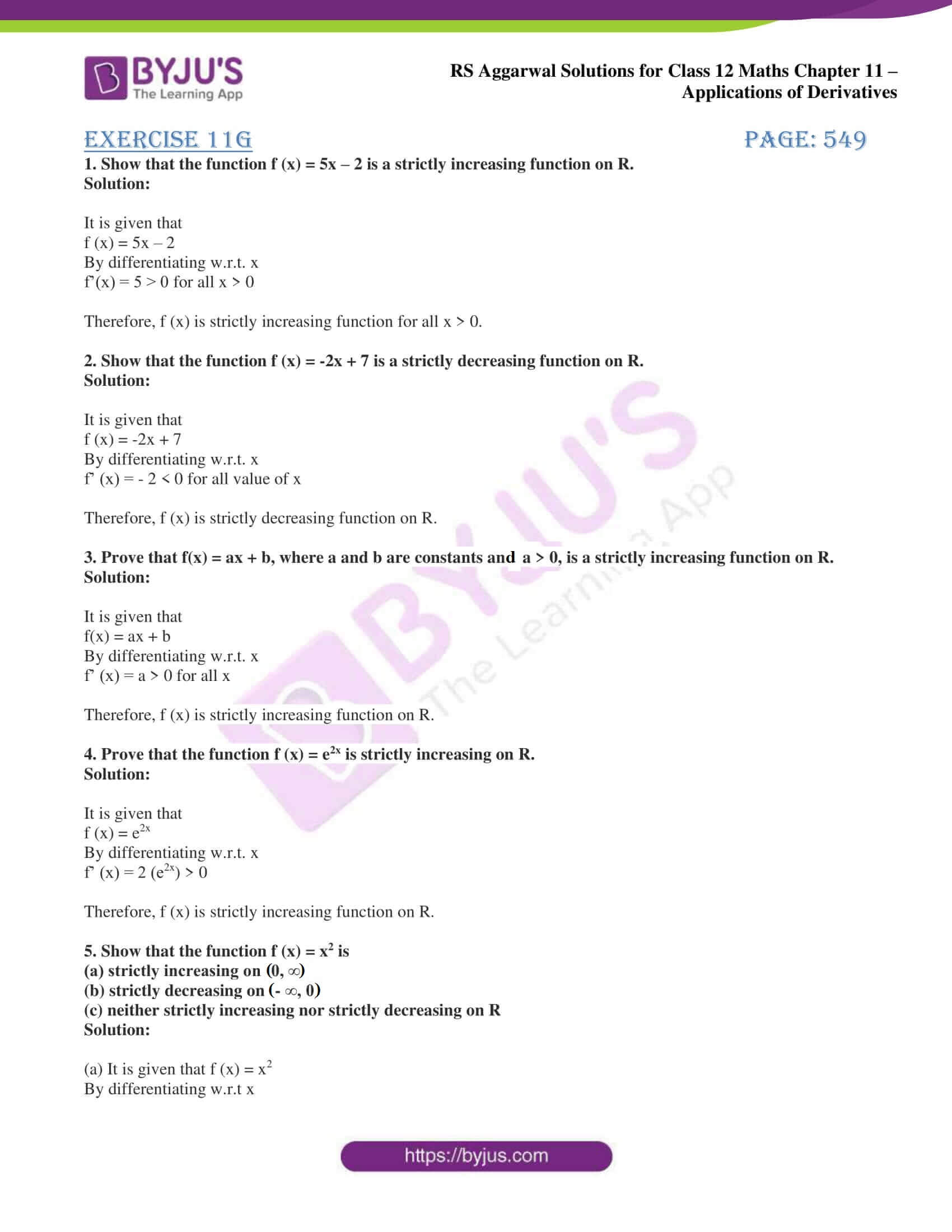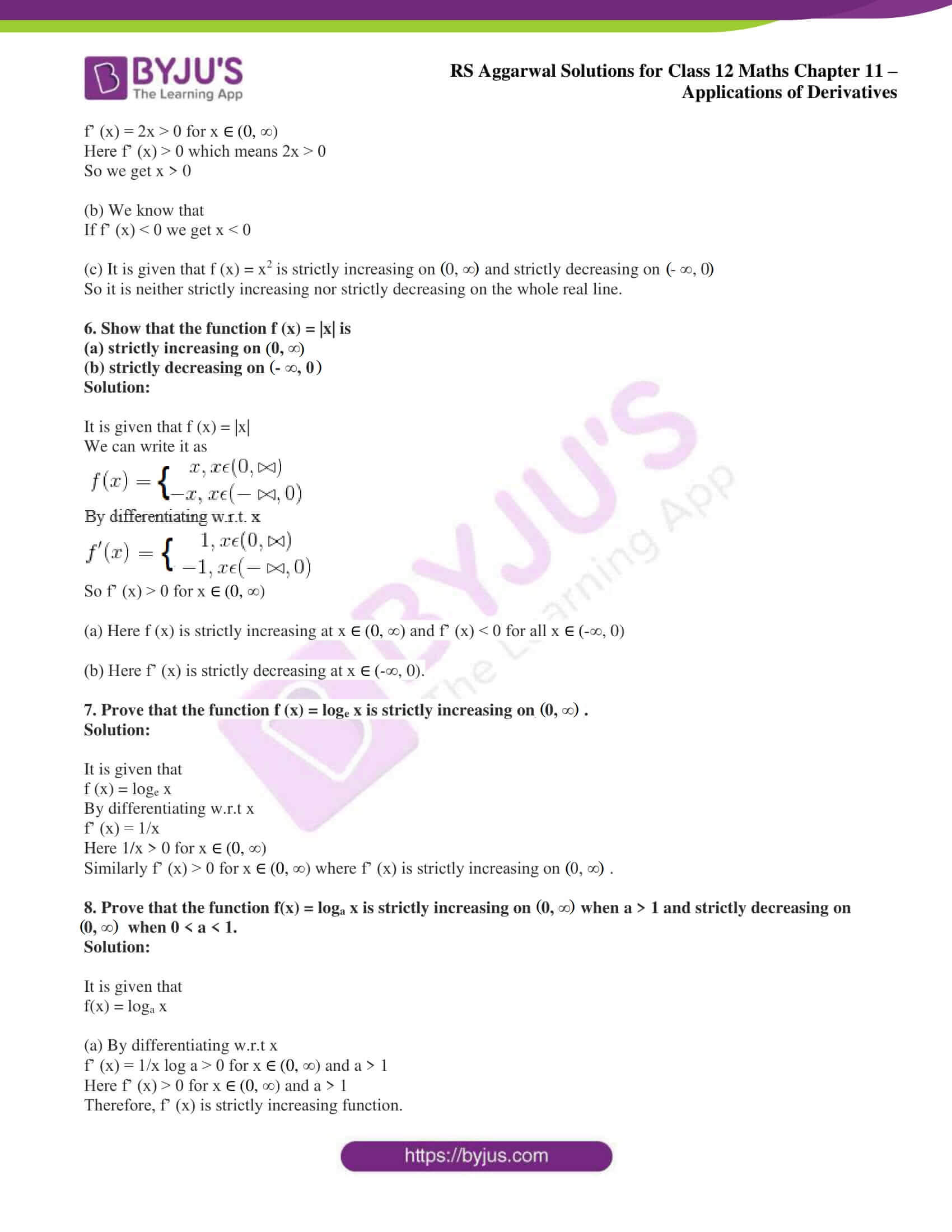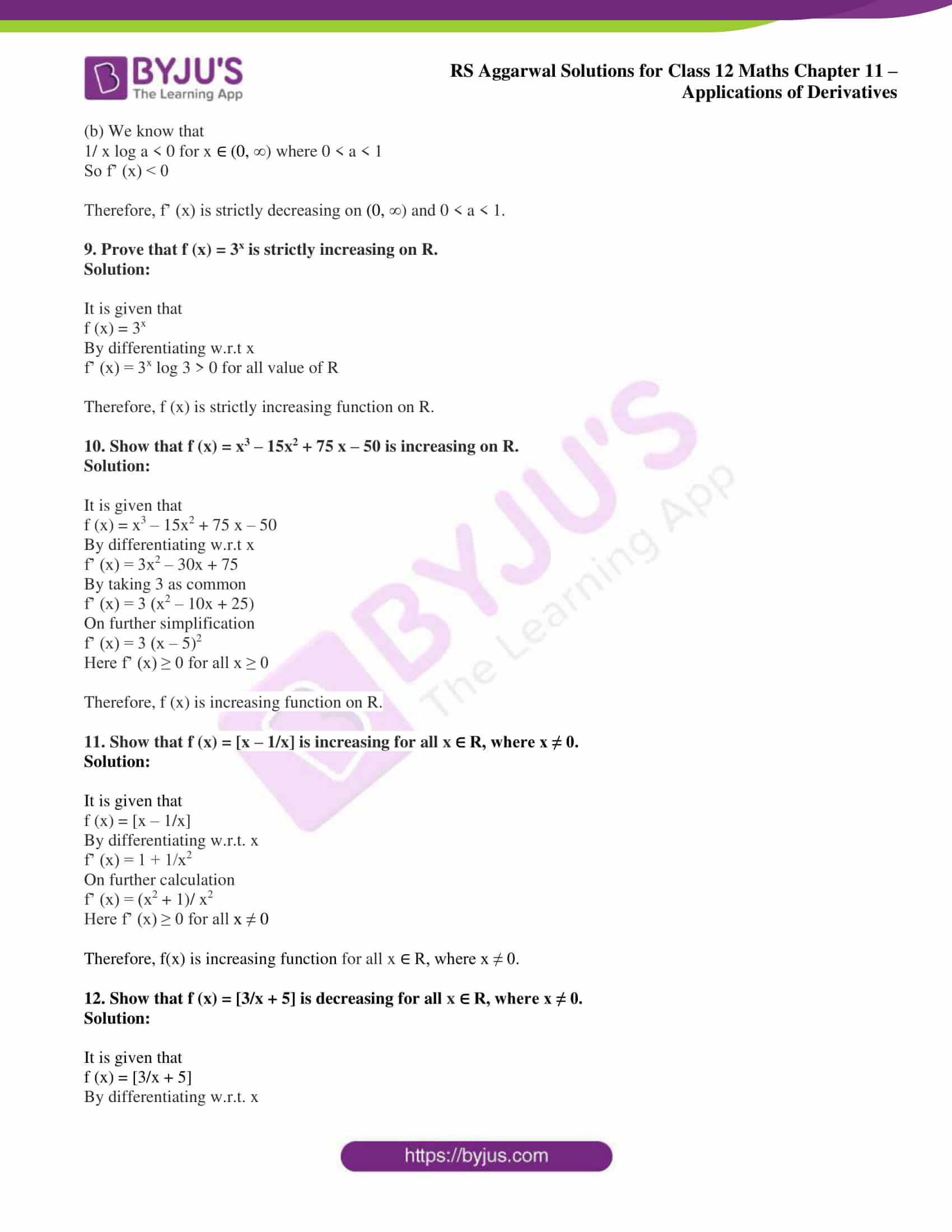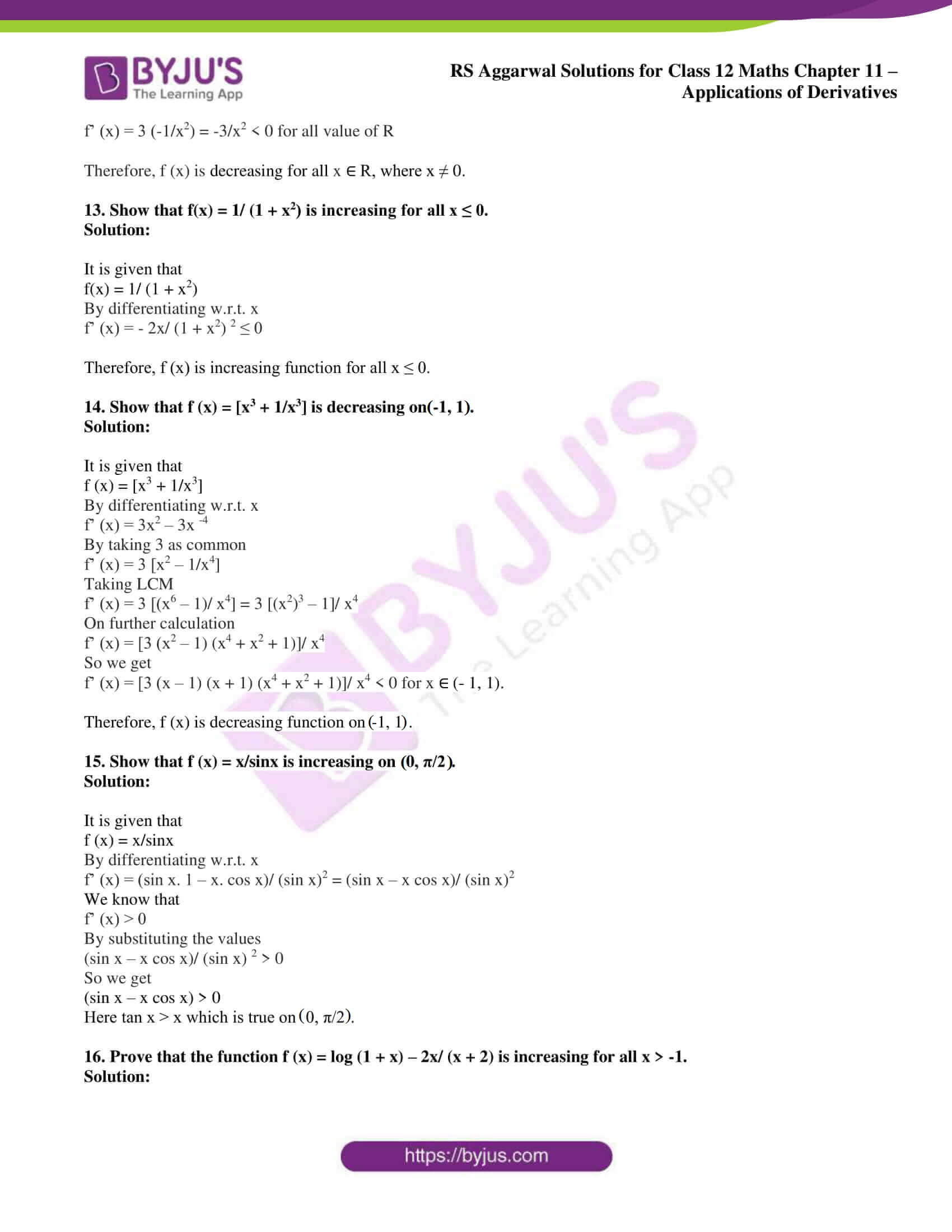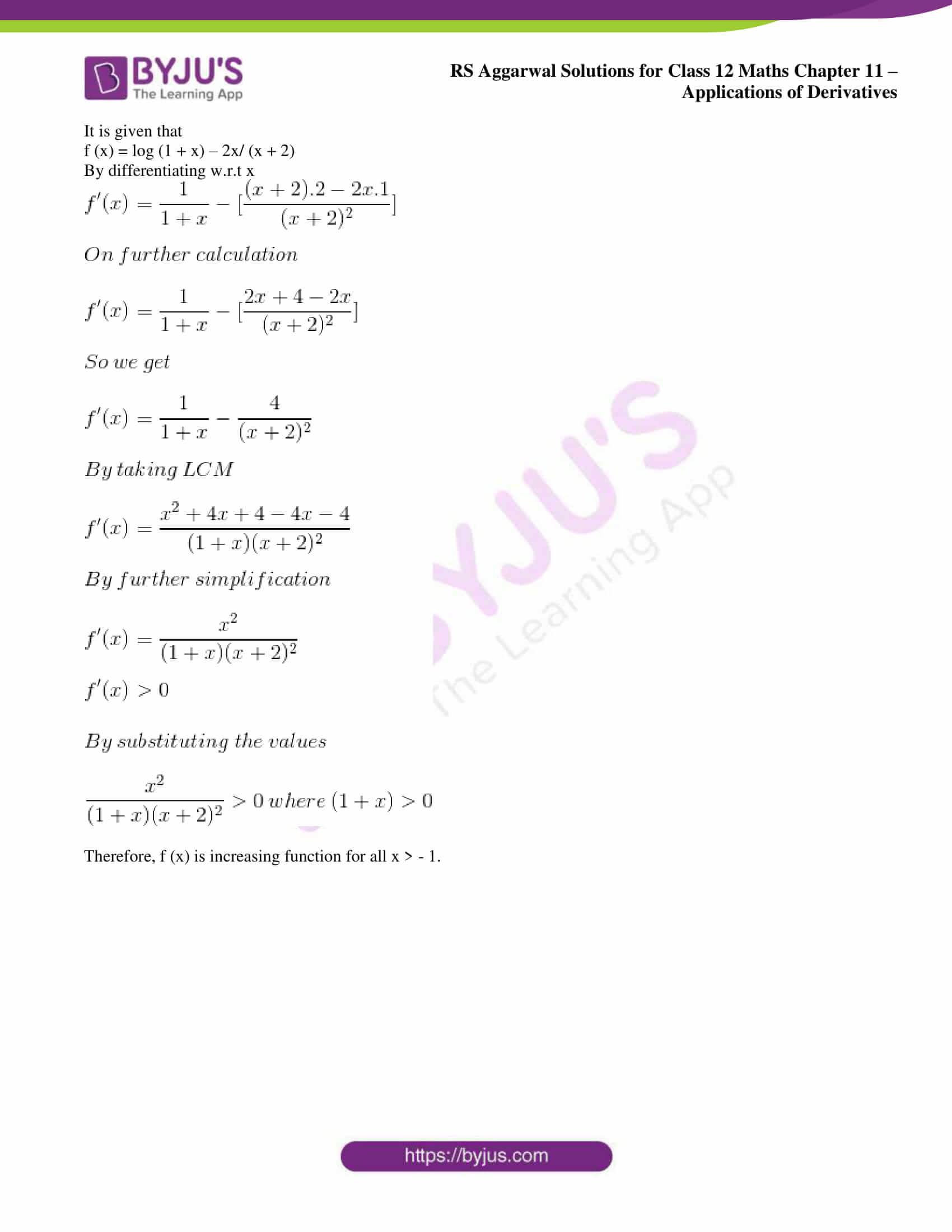### Access other exercise solutions of Class 12 Maths Chapter 11: Applications of Derivatives

Exercise 11A Solutions

Exercise 11B Solutions

Exercise 11C Solutions

Exercise 11D Solutions

Exercise 11E Solutions

Exercise 11F Solutions

Exercise 11H Solutions

### Access RS Aggarwal Solutions for Class 12 Maths Chapter 11: Applications of Derivatives Exercise 11G

1. Show that the function f (x) = 5x – 2 is a strictly increasing function on R.

Solution:

It is given that

f (x) = 5x – 2

By differentiating w.r.t. x

f’(x) = 5 > 0 for all x > 0

Therefore, f (x) is strictly increasing function for all x > 0.

2. Show that the function f (x) = -2x + 7 is a strictly decreasing function on R.

Solution:

It is given that

f (x) = -2x + 7

By differentiating w.r.t. x

f’ (x) = – 2 < 0 for all value of x

Therefore, f (x) is strictly decreasing function on R.

3. Prove that f(x) = ax + b, where a and b are constants and a > 0, is a strictly increasing function on R.

Solution:

It is given that

f(x) = ax + b

By differentiating w.r.t. x

f’ (x) = a > 0 for all x

Therefore, f (x) is strictly increasing function on R.

4. Prove that the function f (x) = e2x is strictly increasing on R.

Solution:

It is given that

f (x) = e2x

By differentiating w.r.t. x

f’ (x) = 2 (e2x) > 0

Therefore, f (x) is strictly increasing function on R.

5. Show that the function f (x) = x2 is

(a) strictly increasing on (0, ∞)

(b) strictly decreasing on (- ∞, 0)

(c) neither strictly increasing nor strictly decreasing on R

Solution:

(a) It is given that f (x) = x2

By differentiating w.r.t x

f’ (x) = 2x > 0 for x ∈ (0, ∞)

Here f’ (x) > 0 which means 2x > 0

So we get x > 0

(b) We know that

If f’ (x) < 0 we get x < 0

(c) It is given that f (x) = x2 is strictly increasing on (0, ∞) and strictly decreasing on (- ∞, 0)

So it is neither strictly increasing nor strictly decreasing on the whole real line.

6. Show that the function f (x) = |x| is

(a) strictly increasing on (0, ∞)

(b) strictly decreasing on (- ∞, 0)

Solution:

It is given that f (x) = |x|

We can write it as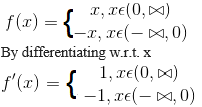So f’ (x) > 0 for x ∈ (0, ∞)

(a) Here f (x) is strictly increasing at x ∈ (0, ∞) and f’ (x) < 0 for all x ∈ (-∞, 0)

(b) Here f’ (x) is strictly decreasing at x ∈ (-∞, 0).

7. Prove that the function f (x) = loge x is strictly increasing on (0, ∞ ).

Solution:

It is given that

f (x) = loge x

By differentiating w.r.t x

f’ (x) = 1/x

Here 1/x > 0 for x ∈ (0, ∞)

Similarly f’ (x) > 0 for x ∈ (0, ∞) where f’ (x) is strictly increasing on (0, ∞).

8. Prove that the function f(x) = loga x is strictly increasing on (0, ∞) when a > 1 and strictly decreasing on (0, ∞ ) when 0 < a < 1.

Solution:

It is given that

f(x) = loga x

(a) By differentiating w.r.t x

f’ (x) = 1/x log a > 0 for x ∈ (0, ∞) and a > 1

Here f’ (x) > 0 for x ∈ (0, ∞) and a > 1

Therefore, f’ (x) is strictly increasing function.

(b) We know that

1/ x log a < 0 for x ∈ (0, ∞) where 0 < a < 1

So f’ (x) < 0

Therefore, f’ (x) is strictly decreasing on (0, ∞) and 0 < a < 1.

9. Prove that f (x) = 3x is strictly increasing on R.

Solution:

It is given that

f (x) = 3x

By differentiating w.r.t x

f’ (x) = 3x log 3 > 0 for all value of R

Therefore, f (x) is strictly increasing function on R.

10. Show that f (x) = x3 – 15x2 + 75 x – 50 is increasing on R.

Solution:

It is given that

f (x) = x3 – 15x2 + 75 x – 50

By differentiating w.r.t x

f’ (x) = 3x2 – 30x + 75

By taking 3 as common

f’ (x) = 3 (x2 – 10x + 25)

On further simplification

f’ (x) = 3 (x – 5)2

Here f’ (x) ≥ 0 for all x ≥ 0

Therefore, f (x) is increasing function on R.

11. Show that f (x) = [x – 1/x] is increasing for all x ∈ R, where x ≠ 0.

Solution:

It is given that

f (x) = [x – 1/x]

By differentiating w.r.t. x

f’ (x) = 1 + 1/x2

On further calculation

f’ (x) = (x2 + 1)/ x2

Here f’ (x) ≥ 0 for all x ≠ 0

Therefore, f(x) is increasing function for all x ∈ R, where x ≠ 0.

12. Show that f (x) = [3/x + 5] is decreasing for all x ∈ R, where x ≠ 0.

Solution:

It is given that

f (x) = [3/x + 5]

By differentiating w.r.t. x

f’ (x) = 3 (-1/x2) = -3/x2 < 0 for all value of R

Therefore, f (x) is decreasing for all x ∈ R, where x ≠ 0.

13. Show that f(x) = 1/ (1 + x2) is increasing for all x ≤ 0.

Solution:

It is given that

f(x) = 1/ (1 + x2)

By differentiating w.r.t. x

f’ (x) = – 2x/ (1 + x2) 2 ≤ 0

Therefore, f (x) is increasing function for all x ≤ 0.

14. Show that f (x) = [x3 + 1/x3] is decreasing on (-1, 1).

Solution:

It is given that

f (x) = [x3 + 1/x3]

By differentiating w.r.t. x

f’ (x) = 3x2 – 3x -4

By taking 3 as common

f’ (x) = 3 [x2 – 1/x4]

Taking LCM

f’ (x) = 3 [(x6 – 1)/ x4] = 3 [(x2)3 – 1]/ x4

On further calculation

f’ (x) = [3 (x2 – 1) (x4 + x2 + 1)]/ x4

So we get

f’ (x) = [3 (x – 1) (x + 1) (x4 + x2 + 1)]/ x4 < 0 for x ∈ (- 1, 1).

Therefore, f (x) is decreasing function on (-1, 1).

15. Show that f (x) = x/sinx is increasing on (0, π/2).

Solution:

It is given that

f (x) = x/sinx

By differentiating w.r.t. x

f’ (x) = (sin x. 1 – x. cos x)/ (sin x)2 = (sin x – x cos x)/ (sin x)2

We know that

f’ (x) > 0

By substituting the values

(sin x – x cos x)/ (sin x) 2 > 0

So we get

(sin x – x cos x) > 0

Here tan x > x which is true on (0, π/2).

16. Prove that the function f (x) = log (1 + x) – 2x/ (x + 2) is increasing for all x > -1.

Solution:

It is given that

f (x) = log (1 + x) – 2x/ (x + 2)

By differentiating w.r.t x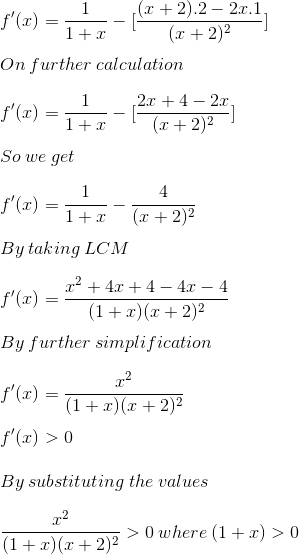Therefore, f (x) is increasing function for all x > – 1.